# Civil Engineering - Surveying

### Exercise :: Surveying - Section 1

16.

If a 30 m chain diverges through a perpendicular distance d from its correct alignment, the error in length, is

 A.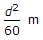B.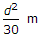C.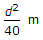D.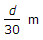E.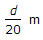.

Explanation:

No answer description available for this question. Let us discuss.

17.

Diopter is the power of a lens having a focal length of

 A. 25 cm B. 50 cm C. 75 cm D. 100 cm E. 125 cm

Explanation:

No answer description available for this question. Let us discuss.

18.

An imaginary line joining the points of equal elevation on the surface of the earth, represents

 A. contour surface B. contour gradient C. contour line D. level line E. none of these.

Explanation:

No answer description available for this question. Let us discuss.

19.

The 'fix' of a plane table station with three known points, is bad if the plane table station lies

 A. in the great triangle B. outside the great triangle C. on the circumference of the circumscribing circle D. none of these.

Explanation:

No answer description available for this question. Let us discuss.

20.

If R is the radius of the main curve, θ the angle of deflection, S the shift and L the length of the transition curve, then, total tangent length of the curve, is

 A. (R - S) tan θ/2 - L/2 B. (R + S) tan θ/2 - L/2 C. (R + S) tan θ/2 + L/2 D. (R - S) tan θ/2 + L/2 E. (R - S) cos θ/2 + L/2